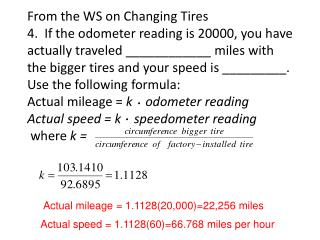DownloadDownload PresentationActual mileage = 1.1128(20,000)=22,256 miles

# Actual mileage = 1.1128(20,000)=22,256 miles

Télécharger la présentation## Actual mileage = 1.1128(20,000)=22,256 miles

- - - - - - - - - - - - - - - - - - - - - - - - - - - E N D - - - - - - - - - - - - - - - - - - - - - - - - - - -
##### Presentation Transcript

1. From the WS on Changing Tires4. If the odometer reading is 20000, you have actually traveled ____________ miles with the bigger tires and your speed is _________.Use the following formula:Actual mileage = k odometer readingActual speed = k speedometer reading where k = Actual mileage = 1.1128(20,000)=22,256 miles Actual speed = 1.1128(60)=66.768 miles per hour

2. What is the percent error in the odometer reading? • Using the odometer reading in the truck equipped with the larger tires, you determine that the gas mileage is 18 miles per gallon. What is your actuall gas mileage in mile per gallon? (22,256 –20,000) / 20,000 = .1128 or 11.28% odometer (66.768 – 60) / 60 = .1128 or .1128 or 11.28 % speedometer The percent error is11.28 % for both. 1.1128(18) = 20.03 miles per gallon

3. If you were driving in the truck with the larger tires and the speedometer showed a speed of 65 miles per hour, could you be ticketed for exceeding the 65-mph speed limit by more that 5mph? More than 10mph? Since 1.1128 times 65 = 72.332, you could be ticketed for speeding more than 5mph but not more than 10mph

4. 8. What is the relationship between the ratio of an actual distance to an odometer distance of 1 mile and the ratio of the circumference of a current tire to the circumference of a factory-installed tire? • On your new small car you replace the factory-installed P185/75R14 tries with slightly larger P205/75R14 tires. Find the missing number in each statement. If your odometer reading is 20000 miles, you have actually traveled _____miles. If you speedometer reading is 60, your actual speed is _____miles per hour. Both of the ratios are equivalent to k. 20948 62.84

5. Diameter P185/75R14= 2 times ht +diameter of rim 2[(.75)(185) ] + 14 = 24.9252 in. The circumference is (pi)(24.9252)=78.3048 in. Diameter of the P205/75R14 : 2[(.75)(205) ] + 14 =26.1063in. The circumference is (pi)(26.1063)=82.0154 in. K = 82.0154/78.3048 = 1.0474

6. Mean, Median, Mode

7. Mean: average Median: number in middle (data must be in order); if there are two numbers in the middle, average them for the median Mode: number that occurs the most times (may have no mode or more than one mode)

8. Find the mean, median, and mode for each set of data. 1. 3,2,3,4,6,6,4,3,2,7

9. 2. stem leaf 5 1, 5, 2, 1, 1, 3 6 4, 0, 1 7 6, 2, 3, 5

10. 3. stem leaf 12 1, 5, 7 13 6, 2, 3, 5, 0 14 8, 1, 0 15 5, 2